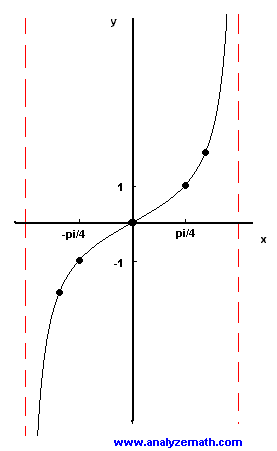# Graphing Tangent Functions

A step by step tutorial on graphing and sketching tangent functions. The graph, domain, range and vertical asymptotes of these functions and other properties are examined.

## Review

Some of the properties of the graph of f(x) = tan(x) are as follows:
1 - The
domain of tan x is the set of all the real numbers except at x = π/2 + n�π , where n is any integer number.
2 - The
range of tanx is the set of all real numbers.
3 - The
vertical asymptotes of the graph of tan x are located at x = π/2 + n�π, where n is any integer.
4 - The
period of tan x is equal to π.

### Example 1

Graph
f( x ) = tan(x)

Over one period.
Solution to Example 1
tan x is undefined for values of x equal to π/2 and -π/2. However we need to understand the behavior of the graph of tan x as x approaches π/2 and -π/2. Let us look at the values of tan x for x close to π/2 such that x is smaller then π/2.
 x π/2-0.5 π/2-0.1 π/2-0.01 π/2-0.001 π/2 tan x 1.8 10.0 100.0 1000.0 undefined
We note that as x approaches π/2 from the left (by values smaller than π/2) tan x increases indefinitely. We say that the graph of tan x has an asymptote at x = π/2. It is represented by a vertical broken red line x = π/2 in the graph below.
We now look at the values of tan x for x close to -π/2 such that x is larger then -π/2.
 x -π/2+0.5 -π/2+0.1 -π/2+0.01 -π/2+0.001 -π/2 tan x -1.8 -10.0 -100.0 -1000.0 undefined
We note that as x approaches -π/2 from the right (by values larger than -π/2) tan x decreases indefinitely. The graph of tan x has an asymptote at x = -π/2. It is represented by a vertical broken red line x = -π/2 in the graph below.
tan x has an asymptotic behavior close to π/2 and -π/2. Using the values of tan x above plus the following values:
tan 0 = 0, tan (π/4) = 1 and tan (-π/4) = -1,
we start by plotting the points (0,0) , (π/4,1) and (-π/4,-1) and the vertical asymptotes.We then draw a smooth curve passing by the points calculated. Close to the vertical asymptotes, the graph either goes upward indefinitely (close to x = π/2 vertical asymptote) and downward indefinitely (close to x = -π/2 vertical asymptote).We now summarize the graphing of tan x as follows:
Step 1: Make a table of values over one period.
 x -π/2 -π/4 -0 π/4 π/2 tan x VA -1.0 0 1.0 VA
where VA means vertical asymptote.
Step 2: Plot the points and the vertical asymptotes.
Step 3: Draw a curve passing through all points and rising or falling vertically along the vertical asymptotes.

### Example 2

Graph function f given by
f( x ) = 2 tan(2 x - π/4)

Over one period.

Solution to Example 2
Let t = 2 x - π/4. Let us make a table over one period (-π/2 , π/2) using the variable t.

 t -π/2 -π/4 -0 π/4 π/2 2 tan t VA -2.0 0 2.0 VA

We now use the relationship between x and t, t = 2 x - π / 4, to find the values of x corresponding to the values of t used in the above table. Solve t = 2 x - π/4 for x.
x = t / 2 + π / 8
A row showing the x values may be added to the above table: These values of x have been found using x = t / 2 + π / 8 found above and the values of t in the table.
 t -π/2 -π/4 -0 π/4 π/2 2 tan t VA -2.0 0.0 2.0 VA x -π/8 0 π/8 2 π/8 3 π/8

We now have the values of the function 2 tan t and the corresponding x values. We have enough information to graph the given function.### Example 3

Graph function f defined by
f( x ) = - tan(x + π/2)

Over one period.
Solution to Example 3
Let t = x + π/2. We first make a table using t over one period.
 t -π/2 -π/4 -0 π/4 π/2 - tan t VA 1.0 0 -1.0 VA

Solve t = x + π/2 for x.
x = t - π / 2
A row showing the x values is added to the above table.
 t -π/2 -π/4 -0 π/4 π/2 - tan t VA 1.0 0.0 -1.0 VA x -π -3π/4 -π/2 - π/4 0

We now have the values of the function - tan t and the corresponding x values.## More References and Links to Graphing

Graphing Functions
Tangent Function. The tangent function f(x) = a tan(b x + c) + d and its properties such as graph, period, phase shift and asymptotes are explored interactively by changing the parameters a, b, c and d using an applet
Free Maths Tutorials and Problems